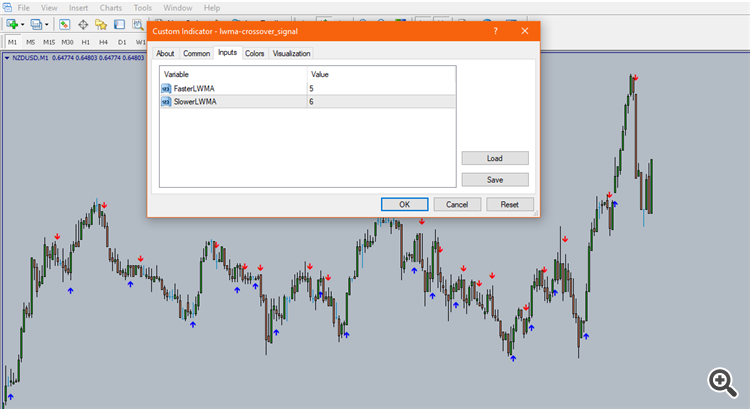# Elite indicators :) - page 1507Moderator
23503

If you want to request code modification, you must attach the mq4 file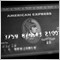715

ValeFX

OK, lets try it out

Austin Passamonte have published something very similar 2 years ago, so it is a kind of a "republishing" of that idea. This one is a version where default values for pivot levels are at 38,61% of the range, but you can easily change it through parameters to the fibo levels Passamonte proposes in his TASC article (0.25,0.50 and 0.75, and then it looks like the picture bellow) or whatever levels you want. If level parameter is set to 0 or less than 0, it will be omitted.
PS: Passamonte in his calculations (published here : CM Dynamic Pivots* TradeStation ) uses a bit different calculation (he actually prefers low) but included that calculation way in the indicator as "AlternativeCalculation" and it looks like this (with levels set to 0.38 and 0.61) It, implicitly, includes levels 25 since what he calls "midHigh" and "midLow" are in fact 25% levels. Since it looks better than the 25,50 and 75% calculation, decided to set those as default parameters
regards

Hi mlanden,

can you update this https://www.mql5.com/en/code/20600

to data below?

```{
Applies to daily, intra-day, and tick bar charts only.
Plots lines at floor trader pivot levels, based on the following
formulae:
Resistance Level 3 = R3 = R2 + YestHigh - YestLow
Resistance Level 2 = R2 = PP + YestHigh - YestLow
Resistance Level 1 = R1 = PP * 2 - YestLow
PivotPoint = PP = ( YestHigh + YestLow + YestClose ) / 3
Support Level 1 = S1 = PP * 2 - YestHigh
Support Level 2 = S2 = PP - YestHigh + YestLow
Support Level 3 = S3 = S2 - YestHigh + YestLow
Input specifies whether R3 and S3 are plotted in addition to the 5
lines at PP, S1, S2, R1, and R2.
}
inputs:
Plot_5or7( 5) ; { if 7, adds plots for S3 and R3 to other 5 lines }
variables:
S1( 0 ),
S2( 0 ),
S3( 0 ),
R1( 0 ),
R2( 0 ),
R3( 0 ),
PP( 0 ),
TodaysHigh( 0 ),
YestHigh( 0 ),
TodaysLow( 0 ),
YestLow( 0 ),
TodaysClose( 0 ),
YestClose( 0 ),
Counter( 0 ) ;
if Date <> Date then
begin
{ increment Counter to be sure enough data is processed - see
comment below }
Counter = Counter + 1 ;
YestHigh = TodaysHigh ;
YestLow = TodaysLow ;
YestClose = Close ;
TodaysHigh = High ;
TodaysLow = Low ;
PP = ( YestHigh + YestLow + YestClose ) / 3 ;
R1 = PP * 2 - YestLow ;
R2 = PP + YestHigh - YestLow ;
R3 = R2 + YestHigh - YestLow ;
S1 = PP * 2 - YestHigh ;
S2 = PP - YestHigh + YestLow ;
S3 = S2 - YestHigh + YestLow ;
end
else
begin
if High > TodaysHigh then
TodaysHigh = High ;
if Low < TodaysLow then
TodaysLow = Low ;
end ;
if Counter >= 2 and BarType < 3 then { if at least one full day’s data
has been processed and chart bar interval is daily, intraday, or tick
bar then plot }
begin
if Plot_5or7 = 7 then
Plot1( R3, “R3” ) ;
Plot2( R2, “R2” ) ;
Plot3( R1, “R1” ) ;
Plot4( PP, “PP” ) ;
Plot5( S1, “S1” ) ;
Plot6( S2, “S2” ) ;
if Plot_5or7 = 7 then
Plot7( S3, “S3” ) ;
end ;```

<Deleted>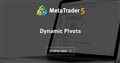Dynamic Pivots
• www.mql5.com
Based on the Austin Passamonte's idea, this indicator calculates intra-day pivots. This one is a version where default values for pivot levels are at 38,61% of the range, but you can easily change it through parameters to the Fibo levels Passamonte proposes in his TASC article...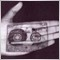178

Hi to everyone here!

Could someone point to OBOS oscillator indicator found in FXCM terminals (non-MQL) please?

Perhaps someone could write it in MQL and make it available for MT5 if possible?

The OBOS oscillator's values are calculated using the following formula:

OBOS Line 1 = EMA{EMA[100 x (WPr-EMA(WPr)) / StdDev(WPr)]}

OBOS Line 2 = EMA(Line 1)

where:
WPr - is the current period weighted price calculated as follows:
WPr=(2 x Close+Low+High) / 4
EMA - is the Exponential Weighted Moving Average of a certain number of periods specified by the Numbers of periods parameter of the OBOS Properties dialog box.
StdDev - is a standard deviation value of WPr calculated over a certain number of periods specified by the Numbers of periods parameter of the OBOS Properties dialog box.

Thanks!

Files:2

jet:

Hello,

Does anyone know where I can find an indicator that takes in price values, which when reached or surpassed will cause the indicator to send a sound alert?11

HI, can someone help me.

This indi is not displaying CCI divergence values... some errors at it.

i've no idea how to fix.

Thanks.

Files:6

Good morning,

Could somebody make this indicator like multisymbol ?

For floating level, select symbo,l one of then.

Thanks.
Files:
velwpr.gif 25 kb14

is not working.why?
Files: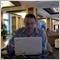Moderator
116027

mohsen_hps1988:
is not working.why?

libSSA.dll file (inside attached zip archive) should be in library folder (indicator is using this file).
Anyway, I am not sure that indicator is working in correct way ... you need to ask coder (probably - in Freelance) to recode this indicator, and without dll file (if possible) -if you really need it.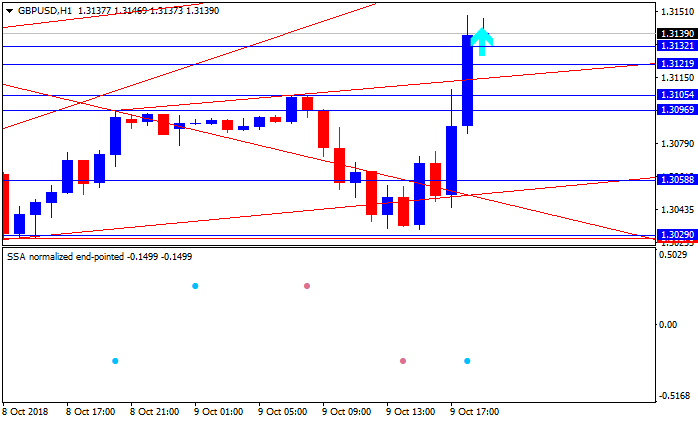Files:
libSSA.zip 5 kb14

Sergey Golubev:

libSSA.dll file (inside attached zip archive) should be in library folder (indicator is using this file).
Anyway, I am not sure that indicator is working in correct way ... you need to ask coder (probably - in Freelance) to recode this indicator, and without dll file (if possible) -if you really need it.

thanks10

It displays the arrow but without any sound alert or message screen.... Please help !